# Simplified Airplane Controller

## Problem

You want to make an airplane controller in 3D, but don’t need a fully accurate flight-simulator.

## Solution

In this recipe, we’re going to make a simplified airplane controller. By “simplified” we mean stripping things down to the basics. We’re looking for the “feel” of flying a plane - one that you can just jump in and start flying effortlessly, with a minimal control scheme.

### Node setup

We’re going to use a `KinematicBody` for this. Since we won’t be simulating actual flight physics (lift, drag, etc.), we don’t need `RigidBody` in this case.

Here’s our model setup: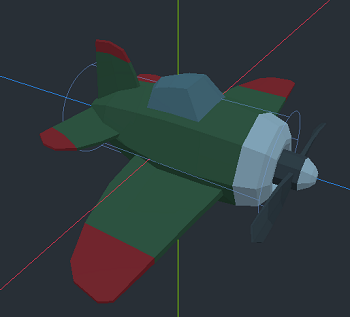We’re using a cylinder for the collision shape, sized to match the plane’s fuselage. This will allow for detecting the ground, which is all we’re concerned with for this demo.

To start the script, let’s look at our plane’s properties:

``````extends KinematicBody

# Can't fly below this speed
var min_flight_speed = 10
# Maximum airspeed
var max_flight_speed = 30
# Turn rate
var turn_speed = 0.75
# Climb/dive rate
var pitch_speed = 0.5
# Wings "autolevel" speed
var level_speed = 3.0
# Throttle change speed
var throttle_delta = 30
# Acceleration/deceleration
var acceleration = 6.0

# Current speed
var forward_speed = 0
# Throttle input speed
var target_speed = 0
# Lets us change behavior when grounded
var grounded = false

var velocity = Vector3.ZERO
var turn_input = 0
var pitch_input = 0
``````

### Controls

We’ll need the following input actions for our controls. We’re using a game controller in this demo, but you can add keyboard inputs as well if you like.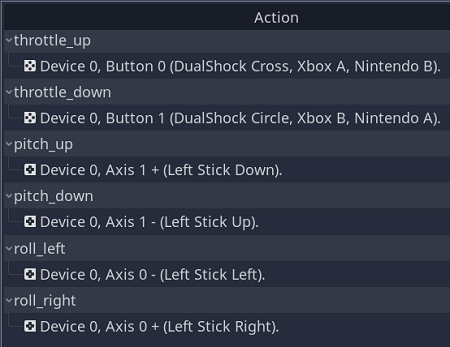This function captures the inputs and sets the input values. Note that increasing/decreasing throttle changes the `target_speed`, not the actual speed.

``````func get_input(delta):
# Throttle input
if Input.is_action_pressed("throttle_up"):
target_speed = min(forward_speed + throttle_delta * delta, max_flight_speed)
if Input.is_action_pressed("throttle_down"):
var limit = 0 if grounded else min_flight_speed
target_speed = max(forward_speed - throttle_delta * delta, limit)
# Turn (roll/yaw) input
turn_input = 0
turn_input -= Input.get_action_strength("roll_right")
turn_input += Input.get_action_strength("roll_left")
# Pitch (climb/dive) input
pitch_input = 0
pitch_input -= Input.get_action_strength("pitch_down")
pitch_input += Input.get_action_strength("pitch_up")
``````

### Movement

Movement happens in `_physics_process()`, first lerping the speed towards the target speed and then using `move_and_slide()`:

``````func _physics_process(delta):
# Accelerate/decelerate
forward_speed = lerp(forward_speed, target_speed, acceleration * delta)
# Movement is always forward
velocity = -transform.basis.z * forward_speed
velocity = move_and_slide(velocity, Vector3.UP)
``````

To test, add the plane to a test scene (don’t forget a `Camera`). Press the `"throttle_up"` input and you should see the plane accelerate forward.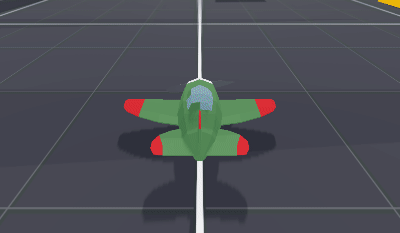Next, let’s handle changing the pitch of the plane. Add this right after calling `get_input()`:

``````transform.basis = transform.basis.rotated(transform.basis.x, pitch_input * pitch_speed * delta)
``````

Run the scene again and try pitching up and down: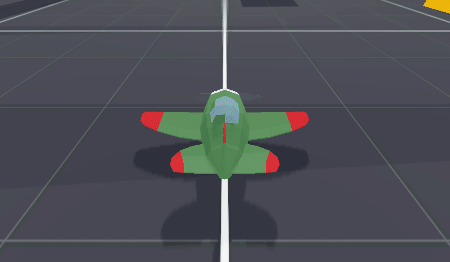After that, add the following for the turn input:

``````transform.basis = transform.basis.rotated(Vector3.UP, turn_input * turn_speed * delta)
``````Notice that while the plane turns, it doesn’t really look natural. Airplanes bank when they turn, so let’s animate that by changing the rotation of the mesh:

``````\$Mesh/Body.rotation.y = lerp(\$Mesh/Body.rotation.y, turn_input, level_speed * delta)
``````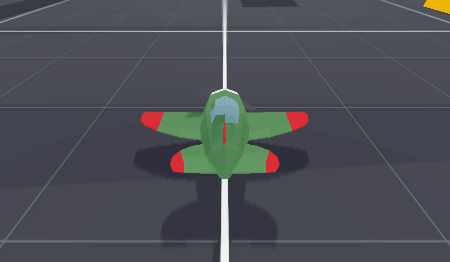The amount of roll is related to the `turn_input` so a shallow turn banks less. Going straight will “auto” level the plane.

That’s it! You now have the basic flying controls working correctly, and it should feel comfortable and natural to fly around. Try adjusting the various properties to see how they affect the movement.

### Landing/taking off

While the above is fine for flying, it doesn’t handle the ground very well. Here, we’ll handle the ground using a simplistic approach (by “simplistic”, we mean doing so in a very basic way - you’ll probably want to expand on it depending on what your game may need).

First, we’ll want to distinguish between being on the ground and being in the air. On the ground, we can slow down to 0; in the air, we must maintain the minimum airspeed. Also, when on the ground, we won’t bank when turning (we don’t want our wings digging into the ground!).

``````func _physics_process(delta):
get_input(delta)
transform.basis = transform.basis.rotated(transform.basis.x, pitch_input * pitch_speed * delta)
transform.basis = transform.basis.rotated(Vector3.UP, turn_input * turn_speed * delta)
# If on the ground, don't roll the body
if grounded:
\$Mesh/Body.rotation.y = 0
else:
\$Mesh/Body.rotation.y = lerp(\$Mesh/Body.rotation.y, turn_input, level_speed * delta)
forward_speed = lerp(forward_speed, target_speed, acceleration * delta)
velocity = -transform.basis.z * forward_speed
# Handle landing/taking off
if is_on_floor():
if not grounded:
rotation.x = 0
velocity.y -= 1
grounded = true
else:
grounded = false

velocity = move_and_slide(velocity, Vector3.UP)
``````

Note that while grounded, we’re setting `velocity.y` to keep us “stuck” to the ground. Alternatively, this could be done via `move_and_slide_with_snap()`.

Meanwhile, in the `get_input()` function, we’ll also take into account `grounded` when throttling down and when pitching down, and by only allowing takeoff if above `min_flight_speed`:

``````func get_input(delta):
if Input.is_action_pressed("throttle_up"):
target_speed = min(forward_speed + throttle_delta * delta, max_flight_speed)
if Input.is_action_pressed("throttle_down"):
var limit = 0 if grounded else min_flight_speed
target_speed = max(forward_speed - throttle_delta * delta, limit)

turn_input = 0
if forward_speed > 0.5:
turn_input -= Input.get_action_strength("roll_right")
turn_input += Input.get_action_strength("roll_left")

pitch_input = 0
if not grounded:
pitch_input -= Input.get_action_strength("pitch_down")
if forward_speed >= min_flight_speed:
pitch_input += Input.get_action_strength("pitch_up")
``````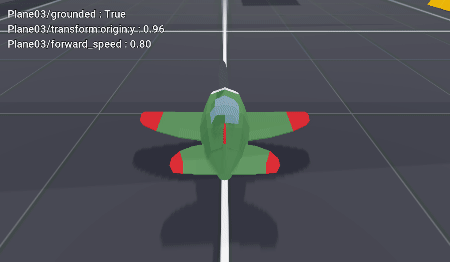### Full script

Here’s the full script:

## Wrapping up

You can adapt this technique to a variety of arcade-style flying games. For example, for mouse control, you could use the `relative` property of `InputEventMouseMotion` to set the pitch and turn input.